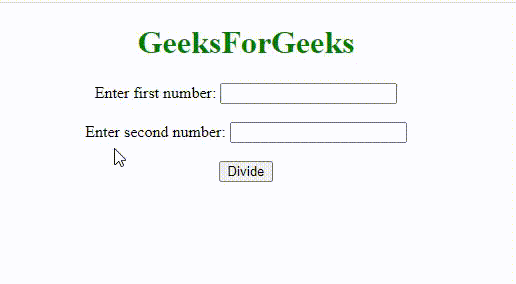Related Articles
How to check first number is divisible by second one in JavaScript ?
• Last Updated : 01 Apr, 2021

Given two numbers and the task is to check the first number is divisible by the second number or not with the help of JavaScript.

Before getting into the coding part, first let us know about the modulo operator and triple equals. To find a number is divisible by another or not, we simply use the reminder operator and if remainder is 0 then it is divisible otherwise not divisible.

Example:

```variable a = 10;
variable b = 5;

if ((a % b) == 0) {
// a is divisible by b
else {
//a is not divisible by b
}
```

We have used “==” operator for comparing the value of expressions. But when it comes to JavaScript we use “===”(Triple equals).

Reason to use Triple equals: The triple equal sign tests for strict equality between two values. Both the type and the value you’re comparing have to be exactly the same.

Examples of strict equality:

```4 === 4
// true (Both numbers, equal values)

'gfg' === 'gfg'
// true (Both Strings, equal values)

54 === '54'
// false (Number compared with String)
```

The double equal tests for loose equality and preforms type coercion. It means we compare two values after converting them to a common type.

Example:

```3 == '3'
// true
```

Hence , we use “===”(Triple equals) in JavaScript rather than “==”(Double equals).

Example: This is the code to check if the first numeric argument is divisible by the second one in JavaScript.

## HTML

 ```<``html``>`` ` `<``body` `style``=``"text-align:center;"``>`` ` `    ``<``h1` `style``=``"color:green;"``>``        ``GeeksForGeeks``    ```` ` `    ``<``p``>``        ``Enter first number:``        ``<``input` `type``=``"text"` `id``=``"txt1"` `name``=``"text1"``><``br``>``        ``<``br``> Enter second number:``        ``<``input` `type``=``"text"` `id``=``"txt2"` `name``=``"text2"``><``br``>``        ``<``br``>``        ``<``button` `onclick``=``"myFunction()"``>Divide``    ```` ` `    ``<``p` `id``=``"demo"` `style="font-size: 20px; ``        ``font-weight: bold;">``    ```` ` `    ``<``script``>``        ``function myFunction() {``            ``var a = Number(document.getElementById("txt1").value);``            ``var b = Number(document.getElementById("txt2").value);``             ` `            ``const isDivisible =``                ``(dividend, divisor) => dividend % divisor === 0;``            ``if (a % b === 0) {``                ``document.getElementById("demo").innerHTML =``                    ``a + " is divisible by " + b;``            ``} else {``                ``document.getElementById("demo").innerHTML =``                    ``a + " is not divisible by " + b;``            ``}``        ``}``    `````` ` ``

Output:Hence, this is how you can check if the first numeric argument is divisible by the second one or not in JavaScript.

My Personal Notes arrow_drop_up